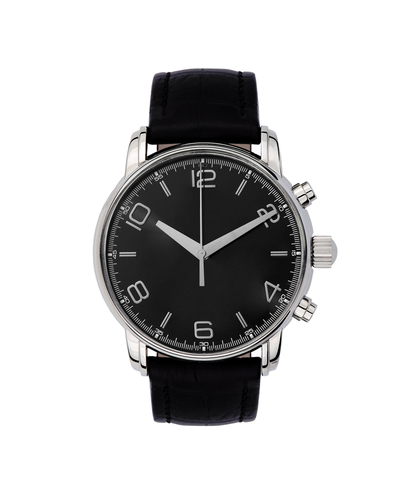# Convert Between 12 Hour and 24 Hour Time

In this worksheet, students will look at converting between 12 hour and 24 hour time, and will solve problems involving time.Key stage:  KS 3

Curriculum topic:   Number

Curriculum subtopic:   Use Standard Units of Measure

Difficulty level:#### Worksheet Overview

What time is 21:35? What about 7:42 am?

We use two main systems for keeping track of time, the 12 hour and 24 hour clocks.How do they work?

The 12 hour clock starts at midnight, and counts from 1 to 12 (midday), before going back to start again, ending up at 12 midnight again.

How do we tell if it's before or after midday?

We use am for before noon and pm for after noon.

The 24 hour clock runs from 00:00 at midnight, all the way to 23:59 at midnight the following day.

If we're using 24 hour format, we don't need am and pm to differentiate.

We do need to make sure that we have two digits on both sides of the dots, so 5:25 am would become 05:25 on the 24 hour clock.So, how do we convert between the two time systems?

To change a 12 hour time to 24 hour

If it's am we don't change the hour, if it's pm we add 12 to the hour.

Example:

What is 7:13 am in 24 hour format?

It's am, so we don't change the hour. Just remember to put a zero before the 7!

7:13 am = 07:13

Remember we don't need the am anymore.

Example:

What is 6:43 pm in 24 hour format?

It's pm, so we add 12 to the hour.

6:43 pm = 18:43And how do we convert a 24 hour time back to 12 hour format?

If the hour is 12 or less, leave it as it is and put am at the end.

If the hour is more than 12, subtract 12 from it and put pm at the end.

Example:

What is 19:57 in 12 hour format?

It's more than 12, so we subtract 12 from 19.

19:57 = 7:57 pm

Example:

What is 11:16 in 12 hour format?

The hour is less than 12, so we leave it as it is and put am at the end.

11:16 = 11:16 am.

Does that make sense?Then let's practise this in some questions.

### What is EdPlace?

We're your National Curriculum aligned online education content provider helping each child succeed in English, maths and science from year 1 to GCSE. With an EdPlace account you’ll be able to track and measure progress, helping each child achieve their best. We build confidence and attainment by personalising each child’s learning at a level that suits them.

Get started